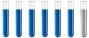## Units of measurement of potential energy

Chemistry and homework help forum.

Organic Chemistry, Analytical Chemistry, Biochemistry, Physical Chemistry, Computational Chemistry, Theoretical Chemistry, High School Chemistry, Colledge Chemistry and University Chemistry Forum.

Share your chemistry ideas, discuss chemical problems, ask for help with scientific chemistry questions, inspire others by your chemistry vision!

Please feel free to start a scientific chemistry discussion here!

Discuss chemistry homework problems with experts!

Ask for help with chemical questions and help others with your chemistry knowledge!

Moderators: expert, ChenBeier, Xen

Dhamnekar Winod
Sr. Staff MemberPosts: 172
Joined: Sat Nov 21, 2020 10:14 am
Location: Mumbai[Bombay],Maharashtra State,India

### Units of measurement of potential energy

Potential energy formula is $$PE=\frac{kq1q2}{r}$$where $$k=\frac{8.99e9J*m}{C^2}$$ How is this k value derived? What is $$\frac{J*m}{C^2}?$$
Any science consists of the following process. 1) See 2)Hear 3)Smell,if needed 4)Taste, if needed 5)Think 6)Understand 7)Inference 8)Take decision [Believe or disbelieve, useful or useless, true or false, cause or effect, any other criteria]
ChenBeier
Distinguished MemberPosts: 798
Joined: Wed Sep 27, 2017 7:25 am
Location: Berlin, Germany

### Re: Units of measurement of potential energy

C = Coulomb = As

Energy Pe has unit J = Joule

So if Pe = k* q1*q2/ r then k = Pe*r/q1*q2 in unit J * m/(As)^2 = J*m/C^2
Dhamnekar Winod
Sr. Staff MemberPosts: 172
Joined: Sat Nov 21, 2020 10:14 am
Location: Mumbai[Bombay],Maharashtra State,India